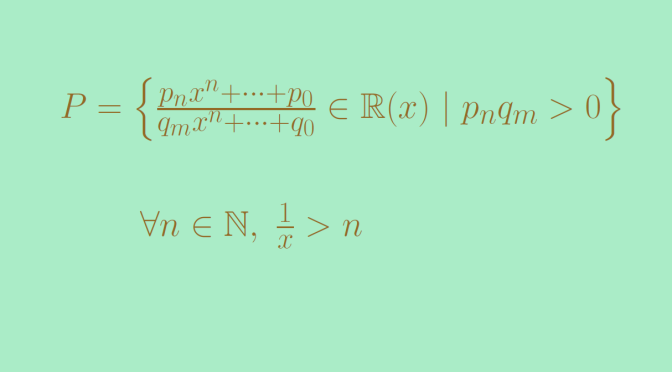A non Archimedean ordered field

Let’s recall that an ordered field $$K$$ is said to be Archimedean if for any $$x \in K$$ there exists a natural number $$n$$ such that $$n > x$$.

The ordered fields $$\mathbb Q$$ or $$\mathbb R$$ are Archimedean. We introduce here the example of an ordered field which is not Archimedean. Let’s consider the field of rational functions
$\mathbb R(x) = \left\{\frac{S(x)}{T(x)} \ | \ S, T \in \mathbb R[x] \right\}$ For $$f(x)=\frac{S(x)}{T(x)} \in \mathbb R(x)$$ we can suppose that the polynomials have a constant polynomial greatest common divisor.

Now we define $$P$$ as the set of elements $$f(x)=\frac{S(x)}{T(x)} \in \mathbb R(x)$$ in which the leading coefficients of $$S$$ and $$T$$ have the same sign.

One can verify that the subset $$P \subset \mathbb R(x)$$ satisfies following two conditions:

ORD 1
Given $$f(x) \in \mathbb R(x)$$, we have either $$f(x) \in P$$, or $$f(x)=0$$, or $$-f(x) \in P$$, and these three possibilities are mutually exclusive. In other words, $$\mathbb R(x)$$ is the disjoint union of $$P$$, $$\{0\}$$ and $$-P$$.
ORD 2
For $$f(x),g(x) \in P$$, $$f(x)+g(x)$$ and $$f(x)g(x)$$ belong to $$P$$.

This means that $$P$$ is a positive cone of $$\mathbb R(x)$$. Hence, $$\mathbb R(x)$$ is ordered by the relation
$f(x) > 0 \Leftrightarrow f(x) \in P.$

Now let’s consider the rational fraction $$h(x)=\frac{1}{x} \in \mathbb R(x)$$. $$h(x)$$ is a positive element, i.e. belongs to $$P$$. And for any $$n \in \mathbb N$$, we have
$h(x)-n=\frac{1}{x}-n=\frac{x-n}{x} \in P$ as the leading coefficients of $$x$$ and $$x-n$$ are both equal to $$1$$. Therefore, we have $$h(x) > n$$ for all $$n \in \mathbb N$$, proving that $$\mathbb R(x)$$ is not Archimedean.